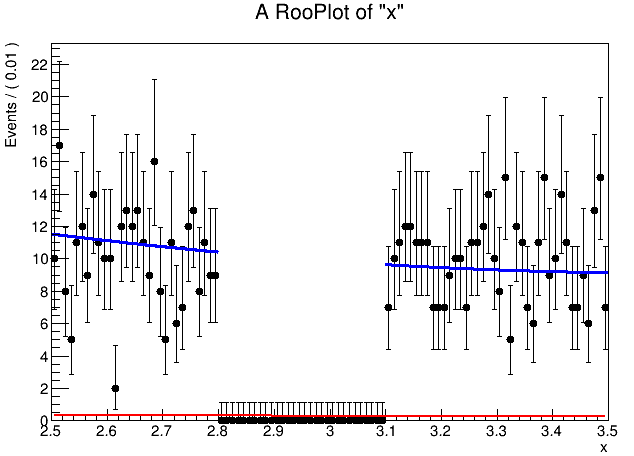# Problem in getting TF1 from extended PDF after range fit

Hello everyone,

I am trying after a fit in sub ranges to get from the extended PDF a TF1 object.
Unfortunately the TF1 I get (red one) is not the correct one (blue one):Am I doing something wrong or is a feature that I am missing?

The corresponding code is:

[code]#ifndef CINT
#include “RooGlobalFunc.h”
#endif

#include “RooRealVar.h”
#include “RooPolynomial.h”
#include “RooPlot.h”
#include “RooNLLVar.h”
#include “RooAbsData.h”
#include “RooAbsPdf.h”
#include “RooAbsArg.h”
#include “RooAbsReal.h”
#include “RooDataSet.h”
#include “RooExtendPdf.h”
#include “RooFitResult.h”
#include “RooArgList.h”
#include “RooMinuit.h”
#include “RooProduct.h”
#include “TF1.h”
#include “TRandom3.h”

using namespace RooFit;

RooDataSet* PolyData(){

RooRealVar x(“x”, “x”, 2.5, 3.5);

RooDataSet *data = new RooDataSet(“data”,“data”,RooArgSet(x)) ;

Int_t i ;
TRandom3 *r = new TRandom3(0);
for (i=0 ; i<1000 ; i++) {
double curVal = r->Uniform(2.5, 3.5);
if (curVal>=2.8 && curVal<=3.1) continue;
x = curVal;
}
data->Print(“v”) ;
cout << endl ;
return data;
}

void newPolyFit(){

RooRealVar x(“x”, “x”, 2.5, 3.5);

RooRealVar c0(“c0”,“c0”, 0, -1e5, 1e4);
RooRealVar c1(“c1”,“c1”, 150, -100, 1e5);
RooPolynomial poly(“poly”,“poly”,x,RooArgList(c0,c1));
RooRealVar npoly(“npoly”,“npoly”, 500, 0, 10e5);
RooExtendPdf epoly(“epoly”,“epoly”,poly, npoly);

RooDataSet* data = PolyData();

x.setRange(“SL”, 2.5, 2.8);
x.setRange(“SU”, 3.1, 3.5);

RooFitResult* result = epoly.fitTo(*data, Save(kTRUE), Range(“SL,SU”),Extended(true));
result->Print(“v”);

RooAbsReal* isum1 = epoly.createIntegral(x, NormSet(x), Range(“SL,SU”));
RooAbsReal* isum2 = epoly.createIntegral(x, NormSet(x));
cout << "Normalization Integral over range[2.5, 2.8] and [3.1, 3.5]: " << isum1->getVal() << endl;
cout << "Normalization Integral over x[2.5, 3.5]: " << isum2->getVal() << endl;

RooPlot* frame = x.frame();
data->plotOn(frame);
epoly.plotOn(frame);
frame->Draw();

RooArgList pars(* epoly.getParameters(RooArgSet(x) ) );

TF1 * f2 = epoly.asTF(RooArgList(x), pars);

f2->Print();
f2->DrawClone(“same”);

cout << f2->Eval(2.5)<< endl;
cout << f2->Integral(2.5, 3.5)<< endl;
cout << f2->Integral(2.5, 2.8)<< endl;
cout << f2->Integral(3.1, 3.5)<< endl;

}[/code]